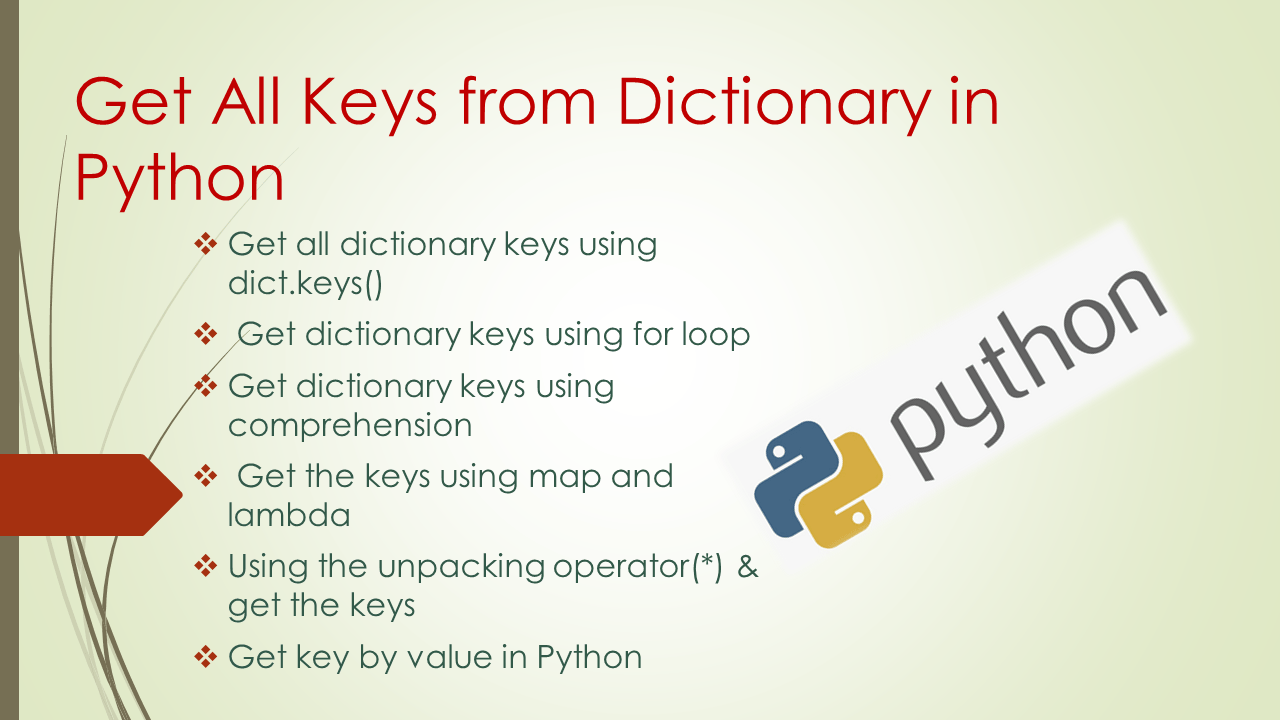# Get All Keys from Dictionary in Python

To get all keys from Dictionary in Python use the keys() method, which returns all keys from the dictionary as a dict_key(). we can also get all keys from a dictionary using various ways in Python. In dictionaries, elements are presented in the form of `key`:`value` pairs and all keys are associated with their corresponding values.

Methods to get all keys from the Dictionary:

1. dict.keys() returns the key in the form of dict_keys([comma separated keys])
2. list(dict.keys()) returns the keys as a list.
3. You can use for loop to iterate the dictionary and get the key from each iteration.
4. Use a list comprehension to get all keys from the dictionary.
5. Finally use map() with lambda to get all keys.

## 1. Quick Examples of Getting All Dictionary Keys

Following are quick examples of getting all dictionary keys in Python.

``````
# Below are the quick examples

# Example 1: Get the keys of dictionary
# using .keys()
keys = my_dict.keys()

# Example 2: Get the keys of dictionary
# using .keys() & list()
keys = list(my_dict.keys())

# Example 3: Get the keys using for loop
for key in my_dict.keys():

# Example 4: Get the keys using dictionary comprehension
keys = [key for key in my_dict]

# Example 5: Use map and lambda get the keys of dict
print(list(map(lambda x: x, my_dict.items())))

# Example 6:  Using * operator get the keys
print([*my_dict])

# Example 7: Get the specified key based on value
keys = [key for key, value in my_dict.items() if value == 'python']
``````

## 2. What is Python Dictionary

A Python dictionary is a key-value collection that is unordered, mutable, and does not allow duplicate keys. Each element in the dictionary is in the form of `key:value` pairs. `Dictionary` elements should be enclosed with `{}` and `key: value` pair separated by commas. The dictionaries are indexed by keys.

Dictionaries are `mutable` hence, you can be added, deleted, and updated their `key:value` pairs. keys are an immutable type such as string, numbers(int, float), and tuple. In case, you add duplicates, it will update the existing key with the new value. Let’s create a Python dictionary,

``````
# Create dictionary
my_dict = {'course':'python','fee':4000,'duration':'60days', 'discount':1200}
print(my_dict)
``````

Yields below output.

## 3. Get All Dictionary Keys using dict.keys()

Using `dict.keys()` method in Python we can get the all keys from the dictionary in the form view object where the order of keys in a list is the same as the insertion order. Let’s call the keys() function on the above-created dictionary.

``````
# Get the keys from dictionary
keys = my_dict.keys()
print(keys)
``````

If you want to get all the keys in the dictionary in the form of a list use the dict.keys() as an argument to the `list()`. The list() function takes the `dict_keys` as an argument and convert it to a list, this will return all keys of the dictionary in the form of a list.

``````
# Get the keys of dictionary as a list
# using .keys() & list()
keys = list(my_dict.keys())
print(keys)

# Output:
# ['course', 'fee', 'duration', 'discount']
``````

## 4. Get Dictionary Keys using for Loop

Use for loop to iterate a python dictionary over keys, by default when you use for loop with dict, it returns a key from the dict for each iteration. For example,

``````
# Get the keys using for loop
for key in my_dict.keys():
print(key)

# Output:
# course
# fee
# duration
# discount
``````

If you wanted to get all keys as a list, use the below example.

``````
# Get the keys using for loop
mylist = []
for key in my_dict.keys():
mylist.append(key)

print(mylist)
``````

### 5. Get Dictionary Keys using Comprehension

Alternatively, using comprehension we can get all the keys of the Python dictionary. Let’s use comprehension(a concise way of creating a new list) and get all keys of the dictionary.

``````
# Get the keys as a list using dictionary comprehension
keys = [key for key in my_dict]
print(keys)

# Output
# ['course', 'fee', 'duration', 'discount']
``````

### 6. Get Keys using Map and lambda

We can also get the dictionary keys using another approach i.e. the combination of a map() function and a lambda function. Let’s use both functions and get our desired output.

``````
# Use map and lambda to get the keys of dict
print(list(map(lambda x: x, my_dict.items())))

# Output:
# ['course', 'fee', 'duration', 'discount']
``````

## 7. Using Unpacking operator(*) & Get the Keys

Using the unpacking operator `'*'` we can get the all keys from the Python dictionary. This operator works with any iterable object and returns a list. Let’s apply ‘*’ operator on the given dictionary.

``````
# Using * operator get the keys
print([*my_dict])

# Output:
# ['course', 'fee', 'duration', 'discount']
``````

## 8. Get Key Based on Value in Python

As you know the dictionary keys are associated with their corresponding values. We can get specified keys of a dictionary based on their corresponding value using list comprehension. For example,

``````
# Get the specified key based on value
keys = [key for key, value in my_dict.items() if value == 'python']
print(keys)

# Output:
# ['course']
``````

## 9. Conclusion

In this Python article, I have explained how to get all keys from the python dictionary using dict.keys() method. Also explained getting keys using map() with lambda, list comprehension, and many other ways.

## References

### Vijetha

Vijetha is an experienced technical writer with a strong command of various programming languages. She has had the opportunity to work extensively with a diverse range of technologies, including Python, Pandas, NumPy, and R. Throughout her career, Vijetha has consistently exhibited a remarkable ability to comprehend intricate technical details and adeptly translate them into accessible and understandable materials.